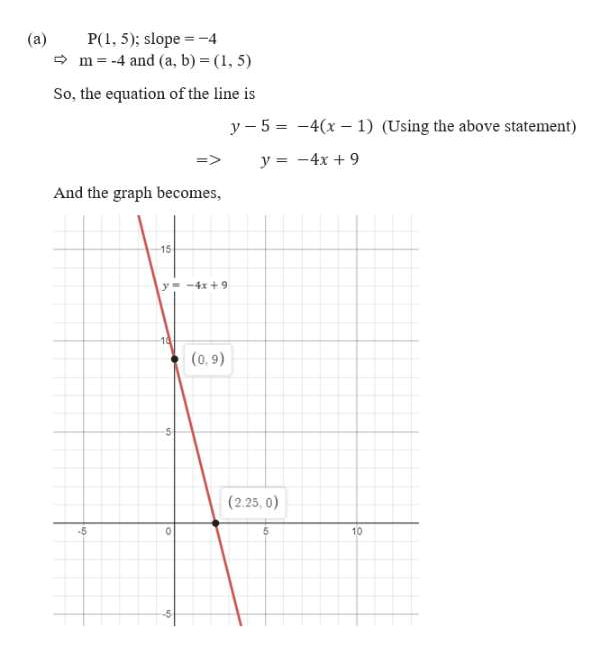# Graph the line that passes through the given point and has the given slope.P(1, 5);slope−4 Graph the line that passes through the given point and has the given slope.P(9, −6);slope -1/3

Question
28 views

Graph the line that passes through the given point and has the given slope.

P(1, 5);
slope
−4

Graph the line that passes through the given point and has the given slope.
P(9, −6);
slope -1/3

check_circle

Step 1

We need to graph the line that passes through the given point and has the given slope. For that reason first we will have to find the linear equation having the given slope and passing through the given points.

Note:

The equation of the line having slope ‘m’ and passing through the point (a, b) is given by y - b = m( x – a ).

Step 2

Here,

...help_outlineImage TranscriptioncloseP( 5); slope -4 m 4 and (a, b) (, 5) (a) So, the equation of the line is y 5 -4(x1) (Using the above statement) y 4x9 And the graph becomes, 15 y-4x +9 (0.9) (2.25, 0) 10 fullscreen

### Want to see the full answer?

See Solution

#### Want to see this answer and more?

Solutions are written by subject experts who are available 24/7. Questions are typically answered within 1 hour.*

See Solution
*Response times may vary by subject and question.
Tagged in
MathAlgebra

### Equations and In-equations